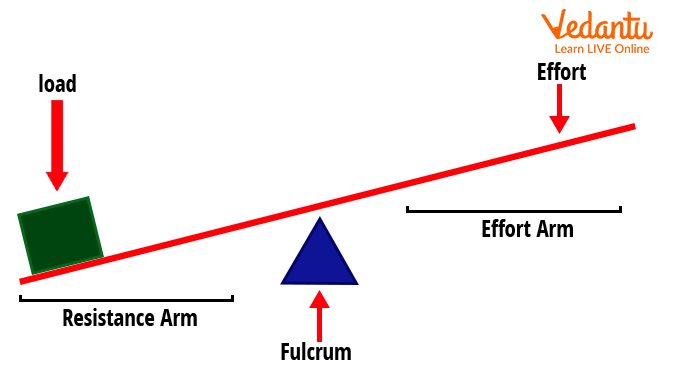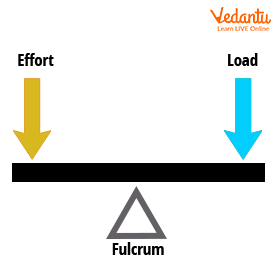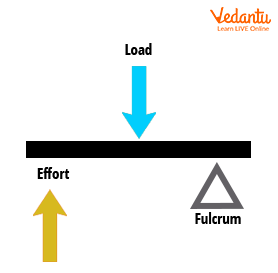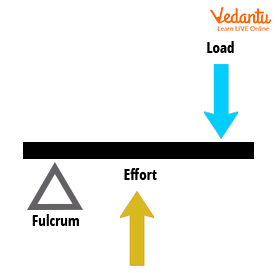Courses
Courses for Kids
Free study material
Free LIVE classes
More

# Levers - JEE Important Topic## Levers – An Introduction

Last updated date: 23rd Mar 2023
Total views: 82.5k
Views today: 1.60k

The devices which were equipped for making the processes like lifting, and moving heavier objects from one position to another position are known as levers. Such a lever helps mankind to perform the task more easily with the application of less force. The very first time when the lever was invented, Archimedes, the ancient scientist, and mathematician was the person behind this invention.

In 5000 BC, the earliest evidence of the lever mechanism was found near East Circa and it was used as a simple balance scale. In our daily life, there are many practical applications where the lever is used. Such practical applications are scissors, bottle openers, shovels, nutcrackers, etc. In this article, the lever system will be discussed along with the lever application, its mechanical advantage, and its working principle. It also discusses its role in biomechanics, and types of the lever with examples.

## What is Lever System?

A lever is a type of simple machine which is used for the amplification of the physical force.  A complete system that comprises a lever mechanism is called a lever system. Simple machines are known for making the process easier and more efficient. In the lever system, there are major components, i.e. rigid beam and a fulcrum. In a lever, the fulcrum is that fixed point about which the beam moves on the application of force at any one end of the beam.

For the proper functioning of the lever, an input force (effort) is applied at one end of the beam for getting an output force (load) at the other end about that pivoted point (fulcrum). The lever is used in various applications which answer those queries like where the lever is used. Examples of the lever are a wheelbarrow, stapler, weighing machine, hockey stick, hammer, etc.

There are five major components of the lever system which are shown below:

• Effort Arm: distance of input force from the fixed point

• Load Arm (Resistance Arm): distance of output force from the fixed point

• Fulcrum: Fixed point of the lever

• Effort: The input force applied on the lever

• Load: The output force obtained after applying effortComponents of Lever System

## Lever Principle

A typical system works on the principle of moments. The principle of moment states that the total clockwise force about a point will be equal to the total anticlockwise force about that same fixed point in a balanced condition.

In the context of the lever system, this principle states that the moment of effort about the fulcrum will be equal to the moment about the load about the fulcrum in the equilibrium condition.

## Types of Levers

There are three types of levers which are shown below:

• First-order lever

• Second-order lever

• Third-order lever

1. ### First Order Lever

The lever of the first order belongs to that category where the fulcrum is located between the load and the effort. In this first order of lever, if the distance between the fulcrum and load is smaller, then less effort is required to move the load for a shorter distance. If the distance between the effort and fulcrum is more, then the load will move for a larger distance. Examples of the lever which belong to the first order are scissors, see-saws, etc.First Order Lever System

1. ### Second-Order Lever

The lever of second-order belongs to that category where the load is located between the fulcrum and the effort. In this second order of lever, if the distance between the fulcrum and load is smaller than the effort, then less amount of effort is required to move the load. If the distance between the effort and load is less than the fulcrum, then more effort is required to move the load. Examples of the lever which belong to the second-order are bottle opener, wheelbarrow, etc.Second Order Lever System

1. ### Third Order Lever

The lever of third-order belongs to that category where the effort is located between the fulcrum and the load. In this third order of lever, if the distance between the fulcrum and load is smaller, then less effort is required to move the load. If the distance between the effort and fulcrum is less, then the load can move a greater distance. Examples of the lever which belongs to the third order are swinging a badminton racket, lifting water from a well, etc.Third-Order Lever System

## Role of Levers in Biomechanics

Role of the levers in biomechanics is to control the movement of the muscles which were attached to the bone of the skeleton and acted as a lever. Just like the lever system, the input force (effort) of the lever system is applied by the muscles of the human body while the output force will be the weight of the body. The point at which the entire process occurred is the point of the joint which acts as a fulcrum.

## Mechanical Advantage of a Lever

The mechanical advantage of a lever is the ratio of the load to the effort. It is the efficiency of the lever for lifting the load on the application of input force. The formula is given as,

Mechanical Advantage = $\frac{Load}{Effort}$

## Application of Lever:

There are various applications of the lever are shown below:

• Using a Screwdriver for Lifting a Lid:

Lifting the lid with the help of a screwdriver is an application of the first-order lever system, in which the tip of the screwdriver fit to the rim of the lid acts as a fulcrum, and the force applied at the end of the screwdriver acts as effort and the opening of the lid act as a load.

• Working with a Broom:

The cleaning of the floor with the help of a broom is an application of a third-class lever system, in which the effort is present in between the fulcrum and load. The hand holding the broom acts as a fulcrum, the effort applied is the force applied for moving the broom, and the brushes of the broom will be the load.

• Nutcracker:

Nutcracker is an application of a second-order lever system, in which the fulcrum is located at the end of the nutcracker, the effort is the force applied to break the things placed in between the cutter, and the result of this applied force is load.

## Conclusion

This article concludes with the conceptual information regarding the lever system. It also answers the queries related to what is a lever, where is a lever used, what are the components of the lever, etc.  It also defines the lever system and its types along with examples. It also describes the components of the lever and elaborates its types based on the order of the lever.

This article covers various examples and types of the lever with examples that answers the queries like where is a lever used. This article covers the principle working of the lever and along with the applications of the lever. The mechanical advantage of the lever depicts the efficiency of the simple machine.

## FAQs on Levers - JEE Important Topic

1. What weightage does the Lever system carry in the JEE exam?

The weightage of the lever system is at a moderate level as it just contains a portion of the mechanics involved in it. This concept uses a simple concept of the principle of moments. According to the JEE Mains point of view, entire mechanics carries high weightage.  But this section has a moderate level of concept from the theoretical point of view. There is a very small variety of numerical in the section of the lever system, as it involves the concept of mechanical advantage. Hence, it is a very less time-consuming topic and an easy chance for scoring marks.

2. What is the requirement of a lever in real life?

The requirement of the lever system is to amplify the amount of applied force for performing any task and obtain a higher output. Those tasks required a large amount of applied force for the completion of tasks. In that case, it is necessary to make certain adjustments so that the least amount of input force will be given maximum output with great efficiency. For this purpose, levers are used in everyday life. It has also been used for enhancing the mechanical advantage of the task.

3. What are the components of the levers system in resemblance to the human body in biomechanics?

In the case of lifting an object by the hand, there are five major components of the lever system will be shown below in resemblance with the human body:

• Effort Arm: It is the length of the muscles inside the human body from the shoulder to the joint of bones.

• Load Arm (Resistance Arm): It is the distance between the joint of the hand and the palm of the hand by which the load will be lifted.

• Fulcrum: The joint of the bones act as a pivot point.

• Effort: It is the muscles power applied by the human hand to lift the weight

• Load: It is the value of the weight which is to be carried.